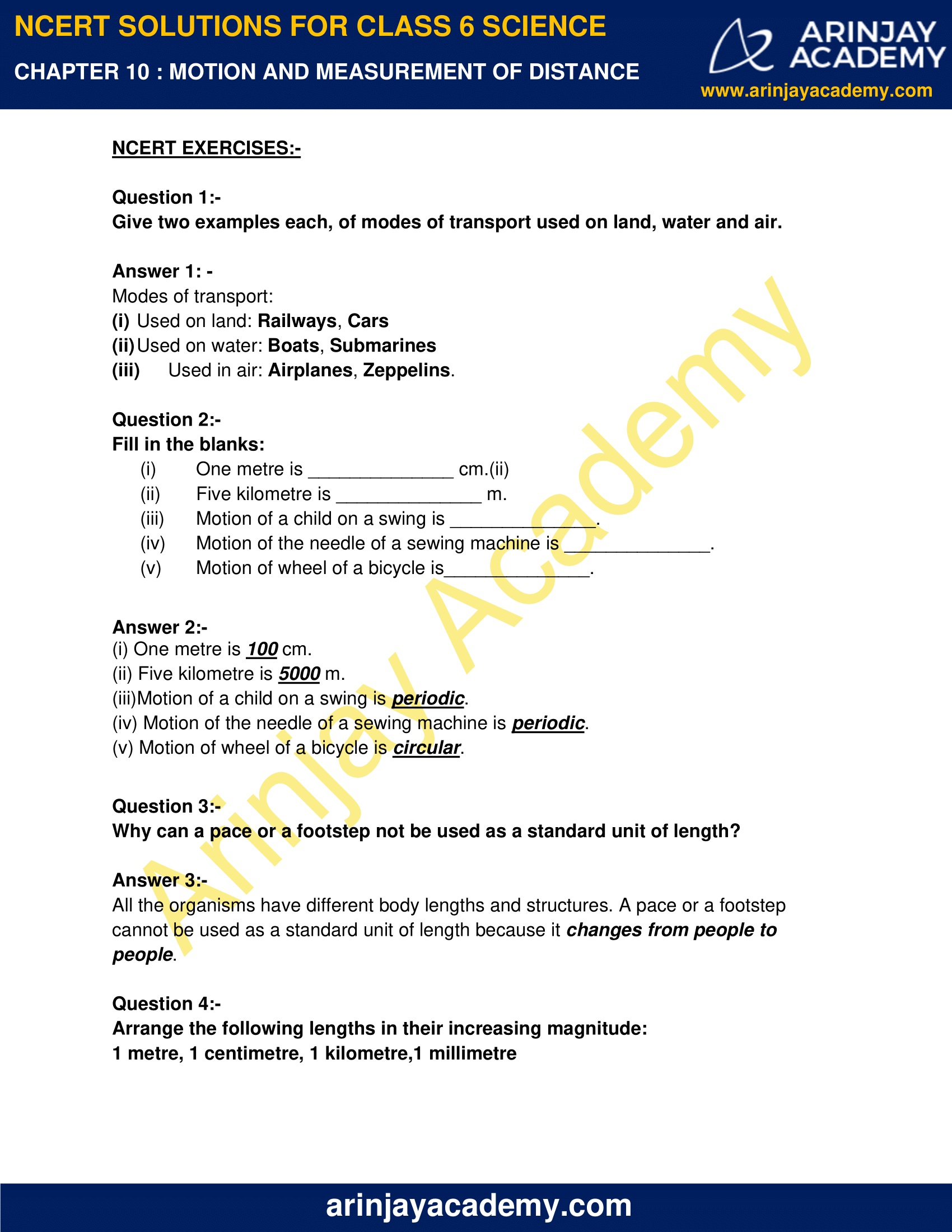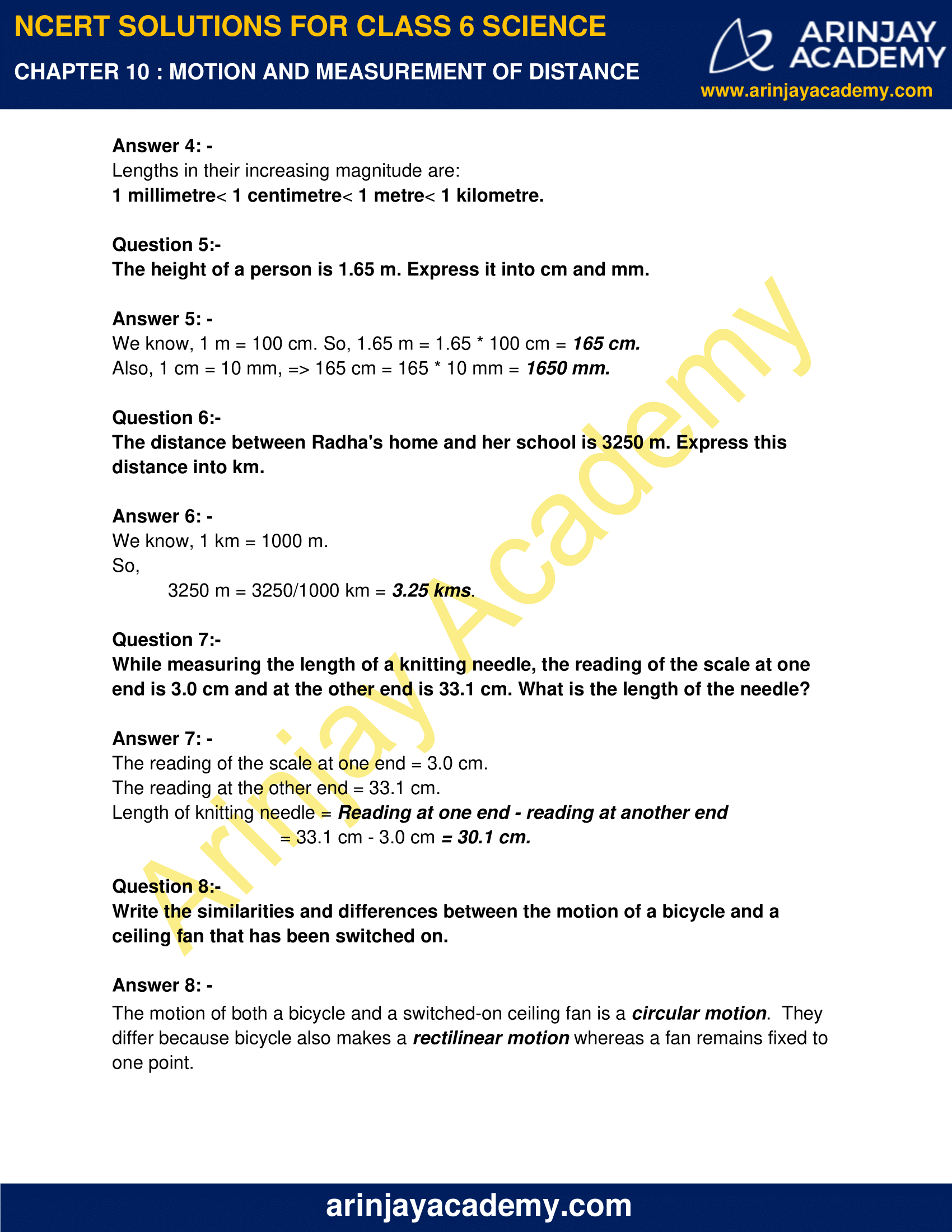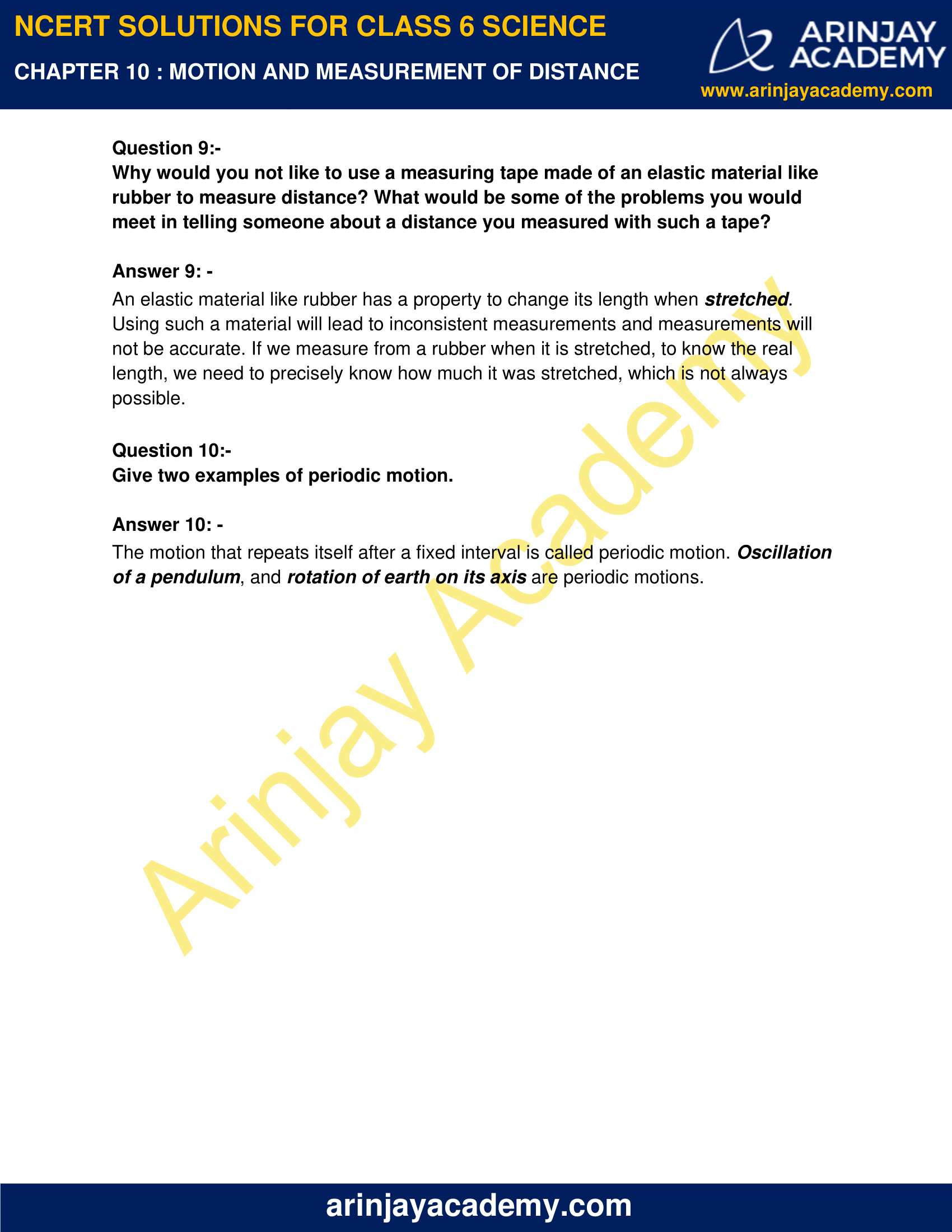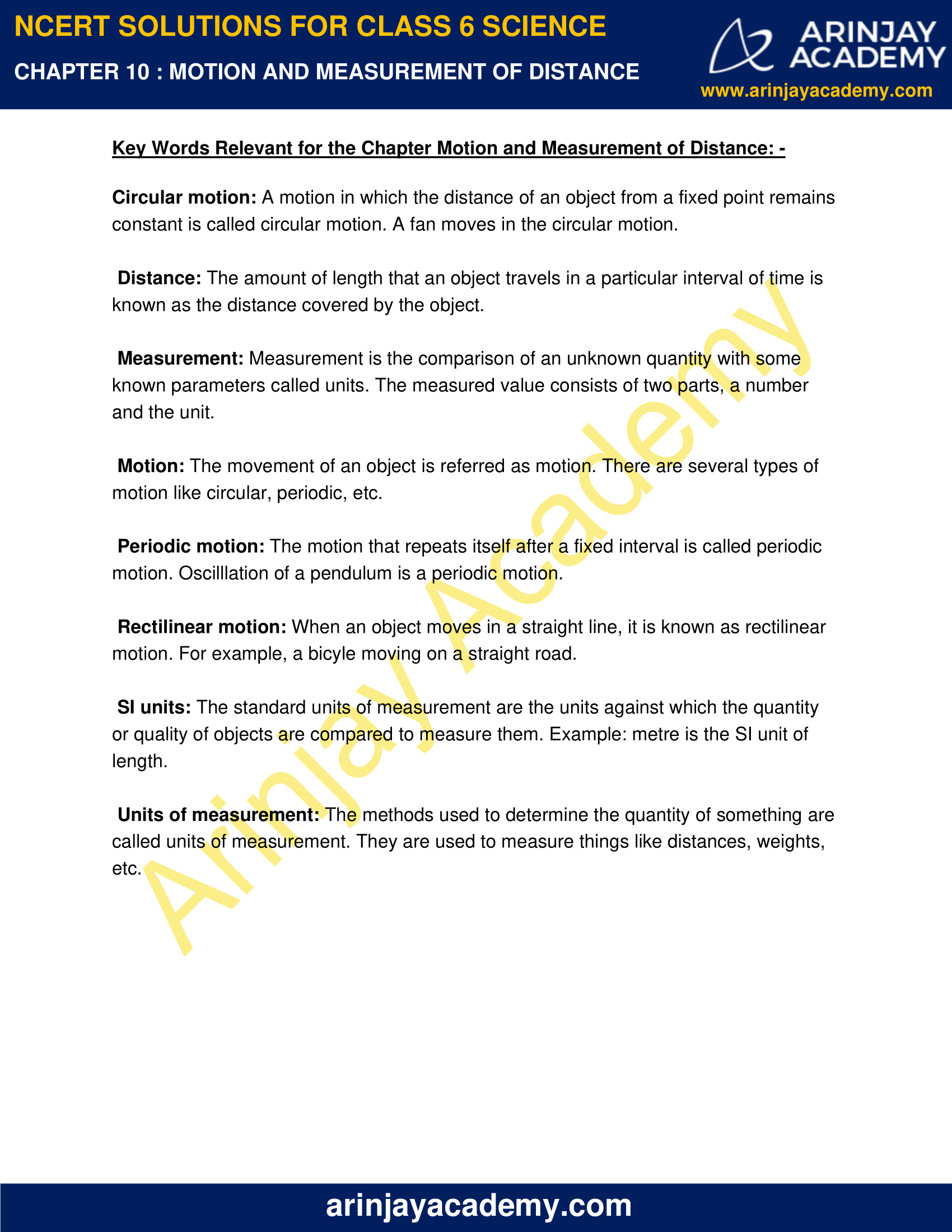# NCERT Solutions for Class 6 Science Chapter 10 – Motion and Measurement of Distance

NCERT Solutions for Class 6 Science Chapter 10 – Motion and Measurement of Distance, contains solutions to various questions in Exercise for Chapter 10.  At the end of the Solutions, all the keywords and Notes which are important to understand NCERT Solutions for Class 6 Science Chapter 10, have been explained in a simple and easy to understand manner. We are providing NCERT Solutions for Class 6 all subjects which can be accessed by clicking here.

NCERT Solutions for Class 6 Science Chapter 10 – Motion and Measurement of Distance – Video Explanation

Long ago people did not have any means of transport. They used to move only on foot and carry goods either on their back or using animals.

Invention of wheel made a great change in modes of transport. But how did people know how far they have travelled?

It is often important to know how far a place is, so that we can have an idea how we are going to reach that place- walk, take a bus or a train, a ship, an aeroplane or even a spacecraft!

## Download NCERT Solutions for Class 6 Science Chapter 10 – Motion and Measurement of Distance

NCERT Solutions for Class 6 Science Chapter 10 – Motion and Measurement of Distance – Solutions to Question 1 to 3NCERT Solutions for Class 6 Science Chapter 10 – Motion and Measurement of Distance – Solutions to Question 4 to 8NCERT Solutions for Class 6 Science Chapter 10 – Motion and Measurement of Distance – Solutions to Question 9 and 10NCERT Solutions for Class 6 Science Chapter 10 – Motion and Measurement of Distance – Definition of the Keywords – Circular motion, Distance, Measurement, Motion, Periodic motion, Rectilinear motion, SI units and Units of measurementScience Chapter 10 Motion and Measurement of Distance, Exercises includes – Questions/ Answers and Fill in the blanks, which helps you to understand the topic covered in the Motion and Measurement of Distance, in a better manner to help you to score good marks in your examinations.

### NCERT Solutions for Class 6 Science Chapter 10 – Motion and Measurement of Distance – NCERT Exercises

Question 1:-

Give two examples each, of modes of transport used on land, water and air.

Modes of transport:

(i) Used on land: Railways, Cars
(ii) Used on water: Boats, Submarines
(iii) Used in air: Airplanes, Zeppelins.

Question 2:-

Fill in the blanks:

(i) One metre is ______________ cm.(ii)
(ii) Five kilometre is ______________ m.
(iii) Motion of a child on a swing is ______________.
(iv) Motion of the needle of a sewing machine is ______________.
(v) Motion of wheel of a bicycle is______________.

(i) One metre is 100 cm.
(ii) Five kilometre is 5000 m.
(iii)Motion of a child on a swing is periodic.
(iv) Motion of the needle of a sewing machine is periodic.
(v) Motion of wheel of a bicycle is circular.

Question 3:-

Why can a pace or a footstep not be used as a standard unit of length?

All the organisms have different body lengths and structures. A pace or a footstep cannot be used as a standard unit of length because it changes from people to people.

Question 4:-

Arrange the following lengths in their increasing magnitude:
1 metre, 1 centimetre, 1 kilometre,1 millimetre

Lengths in their increasing magnitude are:
1 millimetre< 1 centimetre< 1 metre< 1 kilometre.

Question 5:-

The height of a person is 1.65 m. Express it into cm and mm.

We know, 1 m = 100 cm. So, 1.65 m = 1.65 x 100 cm = 165 cm.
Also, 1 cm = 10 mm, => 165 cm = 165 x 10 mm = 1650 mm.

Question 6:-

The distance between Radha’s home and her school is 3250 m. Express this distance into km.

We know, 1 km = 1000 m.
So, 3250 m = 3250/1000 km = 3.25 kms.

Question 7:-

While measuring the length of a knitting needle, the reading of the scale at one end is 3.0 cm and at the other end is 33.1 cm. What is the length of the needle?

The reading of the scale at one end = 3.0 cm.
The reading at the other end = 33.1 cm.
Length of knitting needle
= 33.1 cm – 3.0 cm = 30.1 cm.

Question 8:-

Write the similarities and differences between the motion of a bicycle and a ceiling fan that has been switched on.

The motion of both a bicycle and a switched-on ceiling fan is a circular motion.  They differ because bicycle also makes a rectilinear motion whereas a fan remains fixed to one point.

Question 9:-

Why would you not like to use a measuring tape made of an elastic material like rubber to measure distance? What would be some of the problems you would meet in telling someone about a distance you measured with such a tape?

An elastic material like rubber has a property to change its length when stretched. Using such a material will lead to inconsistent measurements and measurements will not be accurate. If we measure from a rubber when it is stretched, to know the real length, we need to precisely know how much it was stretched, which is not always possible.

Question 10:-

Give two examples of periodic motion.

The motion that repeats itself after a fixed interval is called periodic motion. Oscillation of a pendulum, and rotation of earth on its axis are periodic motions.

### Topics Covered in Science Chapter 10 Motion and Measurement of Distance:-

• STORY OF TRANSPORT
• HOW WIDE IS THIS DESK?
• SOME MEASUREMENTS
• STANDARD UNITS OF MEASUREMENTS
• CORRECT MEASUREMENT OF LENGTH
• MEASURING THE LENGTH OF A CURVED LINE
• MOVING THINGS AROUND US
• TYPES OF MOTION

### Important Terms Relevant to understand NCERT Solutions for Class 6 Science Chapter 10 – Motion and Measurement of Distance: –

Circular motion: A motion in which the distance of an object from a fixed point remains constant is called circular motion. A fan moves in the circular motion.

Distance: The amount of length that an object travels in a particular interval of time is known as the distance covered by the object.

Measurement: Measurement is the comparison of an unknown quantity with some known parameters called units. The measured value consists of two parts, a number and the unit.

Motion: The movement of an object is referred as motion. There are several types of motion like circular, periodic, etc.

Periodic motion: The motion that repeats itself after a fixed interval is called periodic motion. Oscilllation of a pendulum is a periodic motion.

Rectilinear motion: When an object moves in a straight line, it is known as rectilinear motion. For example, a bicyle moving on a straight road.

SI units: The standard units of measurement are the units against which the quantity or quality of objects are compared to measure them. Example: metre is the SI unit of length.

Units of measurement: The methods used to determine the quantity of something are called units of measurement. They are used to measure things like distances, weights, etc.